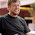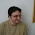Tuesday, March 10, 2009

The Problem with Integer Division

Python's handling of integer division is an example of early mistake with huge consequences. As mentioned earlier, when Python was created, I abandoned the approach to numbers that had been used in ABC. For example, in ABC, when you divided two integers, the result was an exact rational number representing the result. In Python however, integer division truncated the result to an integer.

In my experience, rational numbers didn't pan out as ABC's designers had hoped. A typical experience would be to write a simple program for some business application (say, doing one’s taxes), and find that it was running much slower than expected. After some debugging, the cause would be that internally the program was using rational numbers with thousands of digits of precision to represent values that would be truncated to two or three digits of precision upon printing. This could be easily fixed by starting an addition with an inexact zero, but this was often non-intuitive and hard to debug for beginners.

So with Python, I relied on the other numerical model with which I was familiar, C. C has integers and floating point numbers of various sizes. So, I chose to represent Python integers by a C long (guaranteeing at least 32 bits of precision) and floating point numbers by a C double. I then added an arbitrary precision integer type which I called "long."

The major mistake was that I also borrowed a rule that makes sense in C but not so much in a very-high-level language. For the standard arithmetic operations, including division, the result would always be the same type as the operands. To make matters worse, I initially used another misguided rule that forbade mixed-mode arithmetic, with the aim of making the type implementations independent from each other. So, originally you couldn’t add an int to a float, or even an int to a long. After Python was released publicly, Tim Peters quickly convinced me that this was a really bad idea, and I introduced mixed-mode arithmetic with the usual coercion rules. For example, mixing an int and a long operand would convert the argument of type int to long and return a long result and mixing either with float would convert the int or long argument to float and return a float result..

Unfortunately, the damage was done--integer division returned an integer result. You might be wondering "Why was this so bad?" Was this really just an ado about nothing? Historically, the proposal to change this has had some tough opposition from folks who believed that learning about integer division was one of the more useful "rites of passage" for all programmers. So let me explain the reasons for considering this a design bug.

When you write a function implementing a numeric algorithm (for example, calculating the phase of the moon) you typically expect the arguments to be specified as floating point numbers. However, since Python doesn’t have type declarations, nothing is there to stop a caller from providing you with integer arguments. In a statically typed language, like C, the compiler will coerce the arguments to floats, but Python does no such thing – the algorithm is run with integer values until the wonders of mixed-mode arithmetic produce intermediate results that are floats.

For everything except division, integers behave the same as the corresponding floating point numbers. For example, 1+1 equals 2 just as 1.0+1.0 equals 2.0, and so on. Therefore one can easily be misled to expect that numeric algorithms will behave regardless of whether they execute with integer or floating point arguments. However, when division is involved, and the possibility exists that both operands are integers, the numeric result is silently truncated, essentially inserting a potentially large error into the computation. Although one can write defensive code that coerces all arguments to floats upon entry, this is tedious, and it doesn’t enhance the readability or maintainability of the code. Plus, it prevents the same algorithm from being used with complex arguments (although that may be highly special cases).

Again, all of this is an issue because Python doesn’t coerce arguments automatically to some declared type. Passing an invalid argument, for example a string, is generally caught quickly because few operations accept mixed string/numeric operands (the exception being multiplication). However, passing an integer can cause an answer that is close to the correct answer but has a much larger error – which is difficult to debug or even notice. (This recently happened to me in a program that draws an analog clock – the positions of the hands were calculated incorrectly due to truncation, but the error was barely detectable except at certain times of day.)

Fixing integer division was no easy task due to programs that rely on the behavior of integer truncation. A truncating division operator (//) was added to the language to provide the same functionality. In addition, a mechanism ("from __future__ import division") was introduced by which the new integer division semantics could be enabled.Finally , a command line flag (-Qxxx) was added both to change the behavior and to aid in program conversion. Fortunately, the correct behavior has become the default behavior in Python 3000.

1.It would be very interesting to read that discussion about supporting mixed-mode arithmetic. My Google-fu unearths many interesting historical python-dev discussions, but not this one.

2.i was thinking about this issue the other day for no good reason and came to the conclusion that things like // or /f or /. or however you want to do the syntax should be the way all languages work: it just seems completely crazy now to me to ever have the result implicitly depend on the input types. math doesn't work that way, at least not in my head, which of course might be a confused head. just because i'm dividing two integers and don't want to lose any fractional result parts, i have to do some weird type casting hack? how strange.

maybe i'm just missing something, like it is annoying to implement the different versions of / in languages like C. so maybe this is only doable in modern languages or whatnot.

3.Here's a testimony from the integer division wars: neutral-testimony

4.@Marius: maybe you'll find something if you add "Arthur Siegel" to your query. And/or broaden to include edu-sig or python-list.

5.My favorite example of a buggy program is:

def average(seq):
return sum(sequence) / len(sequence)

This code happily passes some tests:

assert average([1.0, 1.5, 2.0]) == 1.5
assert average([10, 20, 30]) == 20

But, it fails miserably with some inputs:

average([1,2,1,2]) --> 1

6.Guido, as someone who missed out on the earlier days of Python, I'm really enjoying this series. I want to thank you for doing it, and encourage you to keep it going!

- Chris

7.Guido, what's an 'inexact zero'? I dont understand the "This could be easily fixed by starting an addition with an inexact zero" sentence.

is 0 an inexact zero and 0.000 doesnt?

8.An inexact zero is a floating point zero. In ABC, 0 and 0.000 would both give exact zeros (rational numbers); to write an inexact zero you'd have to say ~0 or 0E0.

9.I tried to make a 5.1/1 (5.1 divided by 1) in my Python 2.6/Suse Linux (in console)

To my completely shock I got: 5.0999999999999996

I've tested the same operation with Java, PHP and C.
All worked fine...

I'm still in shock :D

Add this to the fact that I was in the process of praising Python to my team members...

10.Alex, this is only a display problem.

Since Python (just like all other languages you mentioned) uses binary floating point, it cannot represent 5.1 exactly. You will notice that if you just enter 5.1 at the command line it will say the same thing. At the root is a simple rule that "rounds" binary numbers to decimal using 17 digits of precision; the binary float that closest approximates 5.1 will be converted back to decimal using that rule and you will see the unexpected outcome.

It is being fixed in Python 3000. See http://bugs.python.org/issue1580 .

11.Thank you so much for this lucid, concise and interesting account! As a scientist, I really appreciate that the division now returns a float.

12.Spanish translation: here.

13.I´m not sure if this exist, but you could add a optional security mechanism (like "from __future__ import safe_division_check") that fails if a the program try to divide two integers with /. So the programmer can change / for the intended // or cast.

Note: Only a member of this blog may post a comment.